# Geometry Vocabulary Flashcards

Set Details Share
created 4 years ago by mcarver
355 views
Geometry Vocabulary
updated 4 years ago by mcarver
Subjects:
math
Page to share:
Embed this setcancel
COPY
code changes based on your size selection
Size:
X

1

an exact spot in space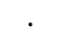Point

2

straight path that continues infinitely in two directions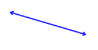Line

3

part of a line with two endpoints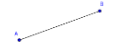Line segment

4

part of a line with one endpoint and one arrow that goes on forever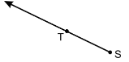Ray

5

an endless flat surface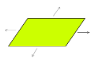Plane

6

lines that never intersect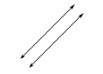parallel

7

lines that cross at a 90 degree angle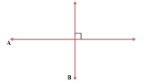perpendicular

8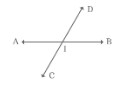lines that pass through the same point

intersecting lines

9

90 degrees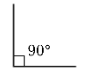a right angle

10

less than 90 degrees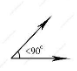acute angle

11

greater than 90 degrees but less than 180 degrees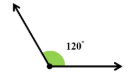obtuse angle

12

180 degrees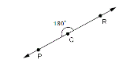a straight angle

13

same exact measurement(s)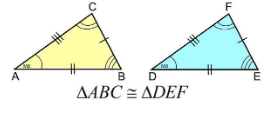congruent

14

Two angles whose sum is 180°

supplementary angles

15

Two angles whose sum is 90°.

Complementary angles

16the pairs of opposite angles made by two intersecting lines (they will be congruent)

vertical angles

17

angles that are right next to each other

18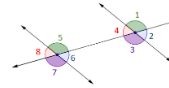two angles in the same relative position at each intersection in a transversal (where a straight lines cross two parallel lines). These angles are congruent.

corresponding angles

19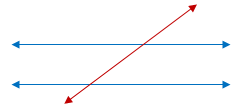parallel lines are intersected by another line, the intersecting line is called a ______________________

transversal

20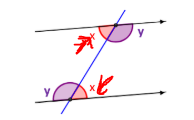Each pair of these angles are inside the parallel lines, and on opposite sides of the transversal (these angles are congruent)

alternate interior angles

21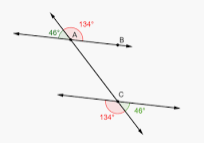a pair of angles on the outer side of each of those two parallel lines but on opposite sides of the transversal (these angles are congruent)

Alternate Exterior Angles

22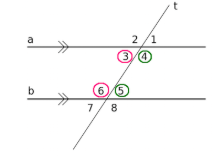the two inside angles on the same side in a traversal will equal _____

180 degrees (same-side interior angles)

23

where two or more line segments or rays meet.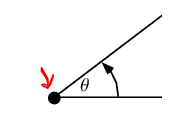vertex

24

a ruler with no markings on it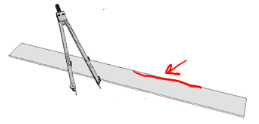straight edge

25

tool used to draw circles and arcs (parts of circles)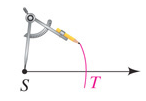compass

26

size of a 2D surface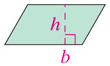area

27

distance around a 2D shape

perimeter

28

line segment with end points on a circle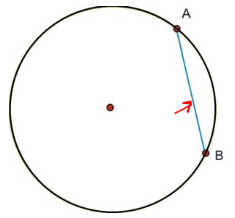chord

29

distance around a circle

circumference

30

Figures with corresponding angles that are equal and corresponding sides that are proportional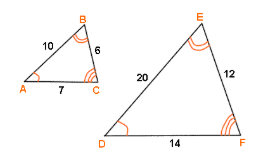similar

31

A chord that passes through the center of the circle from one edge to another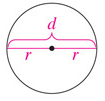diameter

32

A line segment from the center of a circle to the edge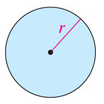33

The total area on all sides (surfaces) of a figure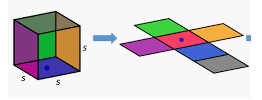surface area

34

The movement of objects in the coordinate plane where image is either similar to or congruent to the pre-image.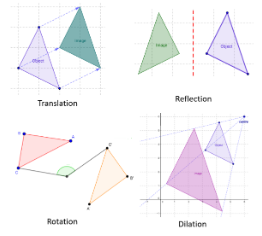transformation

35

A Slide, Each vertex moves the same distance in the same directions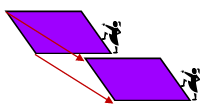translation

36

A turn, Each vertex is rotated the number of degrees around a rotation point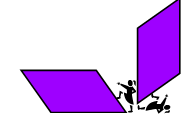rotation

37

A Flip, Each vertex is flipped across a line of reflection to result in a mirror image.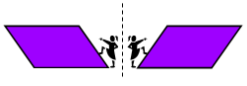reflection

38

a line, segment or ray that goes through the midpoint of a segment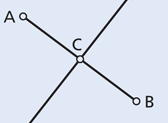bisector

39

A point on a line segment that divides it into two equal parts.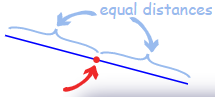midpoint

40

a part of the circumference of a circle or other curve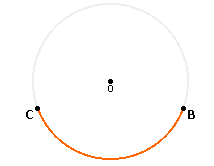arc

41

The amount of space an object takes up.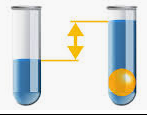volume

42

a quadrilateral with four right angles and 4 congruent sides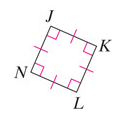square

43

a quadrilateral with four right angles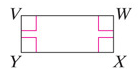rectangle

44

a quadrilateral with four congruent sides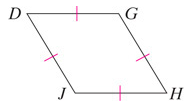rhombus

45

A quadrilateral with two sets of congruent lines that are adjacent to each other.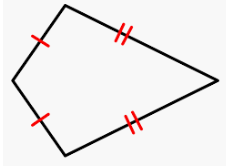kite

46

A triangle with all acute angles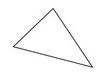acute triange

47

A triangle with one obtuse angle and two acute angles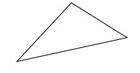obtuse triangle

48

A triangle with one 90 degree angle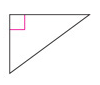right triangle

49

A triangle with all congruent sides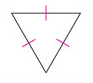equilateral triangle

50

A triangle with at least two congruent sides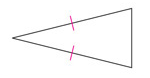isosceles

51

A triangle with no congruent sides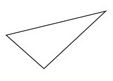scalene triangle

52

quadrilateral with both pairs of opposite sides are parallel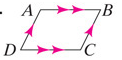parallelogram

53

A closed figure formed by three or more line segments that do not cross.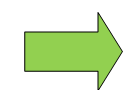polygon

54

four sided polygon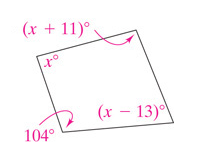four sided polygon

55

A polygon with congruent sides and angles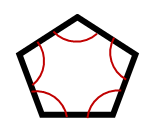regular polygon

56

a quadrilateral with exactly one pair of parallel sides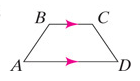trapezoid

57

A set of points in the same plane equal distance from the center.

circle

58

A set of all points in space that are the same distance from the center point.

sphere

59

A 3D object where the rectangular faces are perpendicular to the base shape. The base can be any shape.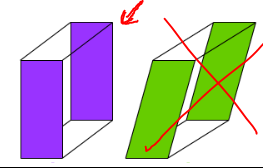right prism

60

3D object with a circular base and one vertex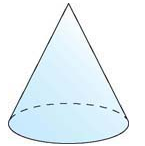cone

61

A prism with triangular faces that meet at one point, a vertex and base that is a polygon.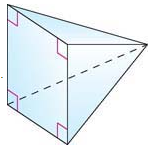pyramid

62

A prism with two congruent parallel bases that are circles.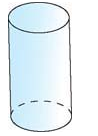cylinder

63

A rectangular prism with faces that are all squares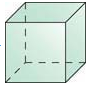cube

64

A line that intersects a curve (or circle) in at least two distinct points.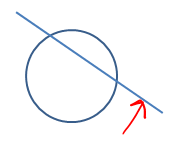Secant

65

A line that touches a curve (or circle) at exactly one point.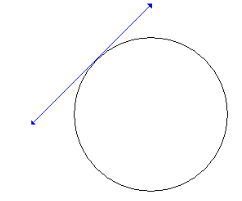Tangent (to a circle)

66

An angle whose vertex is the center O of a circle and whose legs (sides) are radii intersecting the circle in two distinct points A and B.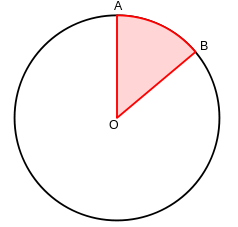Central Angle

67

*Secondary 2 and higher only*

The ratio between the side opposite of an angle and the hypotenuse.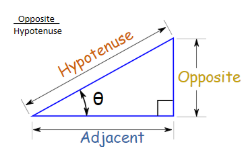*Secondary 2 and higher only*

Sine

68

*Secondary 2 and higher only*

The ratio between the side adjacent to an angle and the hypotenuse.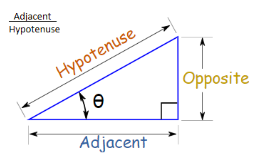*Secondary 2 and higher only*

Cosine

69

*Secondary 2 and higher only*

The ratio between the side opposite of an angle and the side adjacent to the angle.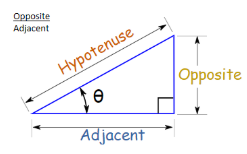*Secondary 2 and higher only*

Tangent (as a triangle ratio)

70

*Secondary 2 and higher only*

Exterior Angles Theorem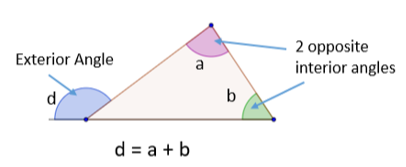*Secondary 2 and higher only*

The exterior angle of a triangle is equal to the sum of the two opposite interior angles